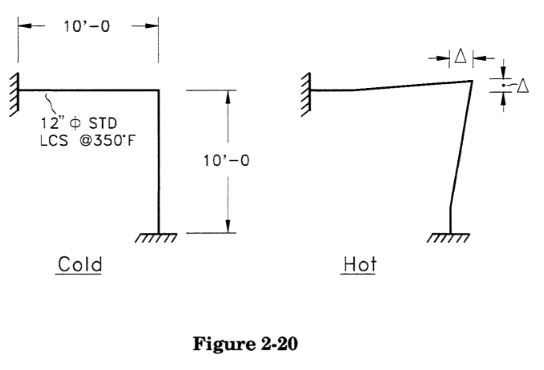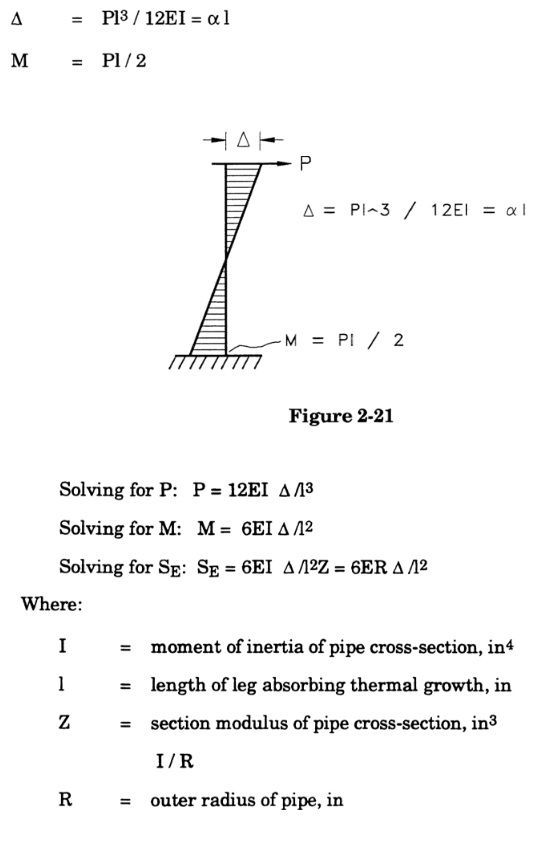top of page
Search

# 2.3.2 Guided Cantilever Method

One proposed means of allowing nearly all of the pipe's free movement (while still holding the system together) is to provide adjacent, perpendicular legs to absorb the thermal growth through bending, as shown in Figure 2-20.Each leg can be modeled as a guided cantilever. According to beam theory:Note that the calculated expansion stress range SE is independent of the wall thickness of the pipe (on a system-wide basis). Therefore, increasing (or decreasing) the pipe wall is usually not an adequate solution to an expansion stress problem. This equation also points out that the stress range decreases with the square of the length of the absorbing leg, so the longer the leg absorbing the displacement, the lower the stress range.

For the configuration shown in Figure 2-20:An expansion stress range of 17,700 psi is normally not a problem, however it must be remembered that this equation did not take into account the stress intensification factor (SIF) at the elbow at the top of the leg. Considering an in-plane stress intensification factor for a long radius bend of typical SIF value of 2.8, this would result in a stress range of about 49,600 psi, which is probably excessive for typical low carbon steel applications. (Note that this value is actually conservative, since the guided cantilever model does not take into account the fact that the junction of the two legs will rotate some under the load, and further neglects the additional flexibility of the elbow.)

Against what do we compare the 49,600 psi stress range? We compare it against SA, which is:For a typical low carbon steel (A106 Grade B, for instance) and a typical piping code (B31.3, for instance), Sc and Sh are both 20,000 psi, giving a conservative value for SA of 30,000 psi (the non-conservative value of SA cannot be calculated without knowing the sustained stress SL at the point of interest).

The expansion stress range can be approximated for any run of pipe using the guided cantilever equation shown above, as long as the displacements to be absorbed are known.